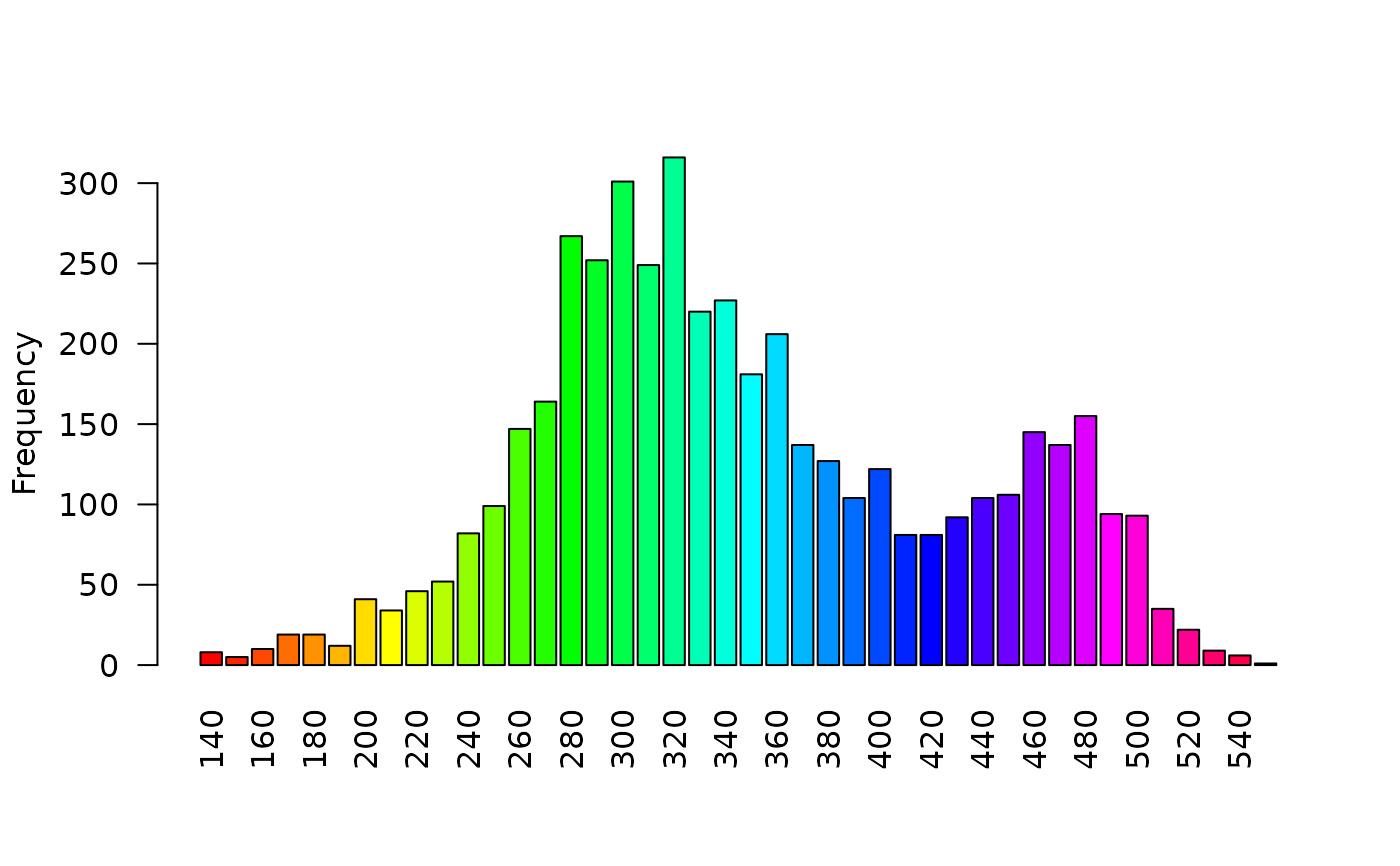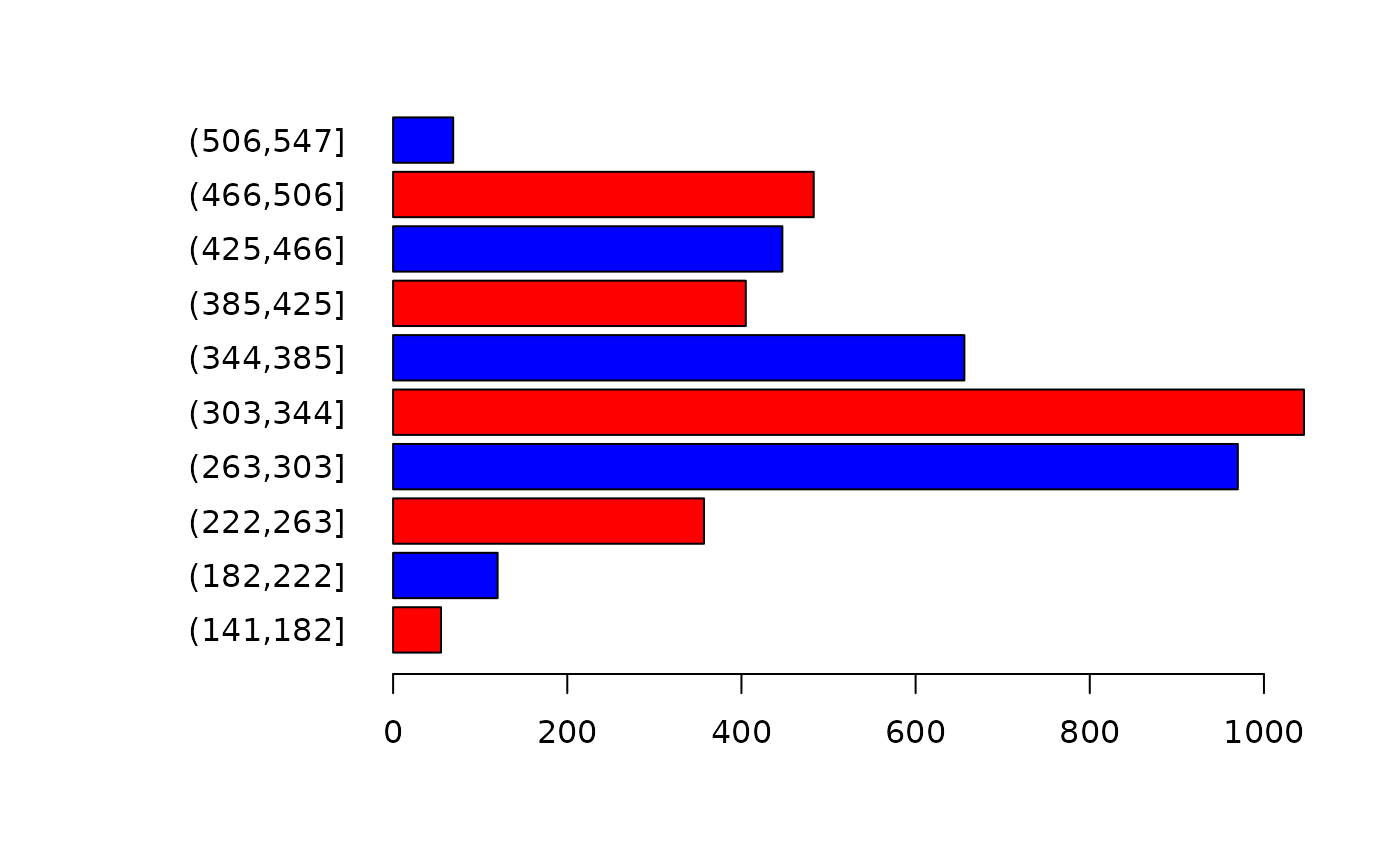Create a barplot of the values of a the first layer of a SpatRaster. For large datasets a regular sample with a size of approximately maxcells is used.

# S4 method for SpatRaster
barplot(height, maxcell=1000000, digits=0, breaks=NULL, col, ...)

## Arguments

height

SpatRaster

maxcell

integer. To regularly subsample very large datasets

digits

integer used to determine how to round the values before tabulating. Set to NULL or to a large number if you do not want any rounding

breaks

breaks used to group the data as in cut

col

a color generating function such as rainbow (the default), or a vector of colors

...

additional arguments for plotting as in barplot

hist, boxplot

## Value

A numeric vector (or matrix, when beside = TRUE) of the coordinates of the bar midpoints, useful for adding to the graph. See barplot

## Examples

f <- system.file("ex/elev.tif", package="terra")
r <- rast(f)
barplot(r, digits=-1, las=2, ylab="Frequency")